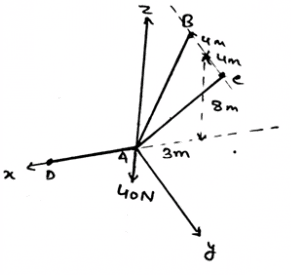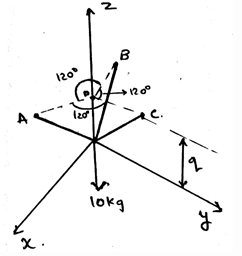Courses

# Test: Three Dimensional Force System

## 10 Questions MCQ Test Engineering Mechanics | Test: Three Dimensional Force System

Description
This mock test of Test: Three Dimensional Force System for Mechanical Engineering helps you for every Mechanical Engineering entrance exam. This contains 10 Multiple Choice Questions for Mechanical Engineering Test: Three Dimensional Force System (mcq) to study with solutions a complete question bank. The solved questions answers in this Test: Three Dimensional Force System quiz give you a good mix of easy questions and tough questions. Mechanical Engineering students definitely take this Test: Three Dimensional Force System exercise for a better result in the exam. You can find other Test: Three Dimensional Force System extra questions, long questions & short questions for Mechanical Engineering on EduRev as well by searching above.
QUESTION: 1

### What is not the condition for the equilibrium in three dimensional system of axis?

Solution:

For the equilibrium in the three dimensional system of axis we have all the conditions true as, ∑Fx=0, ∑Fy=0 and ∑Fz=0. Also we have the summation of the forces equal to zero. Which is not a non-zero value.

QUESTION: 2

### State true or false. We first make equilibrium equations and then the free body diagram and then solve the question?

Solution:

We first make the free body diagram and then we make the equilibrium equations to satisfy the given conditions. This helps us to solve the question easily. As this reduces the part of imagination and increases accuracy too.

QUESTION: 3

### If anybody is tied to three or more ropes, and then is allowed to achieve its equilibrium. Then the equilibrium achieved is achieved w.r.t what?

Solution:

Yes, the equilibrium is being achieved w.r.t the ground. Like the motion, w.r.t ground need be zero. That is the relative velocity of the object or the body must be zero w.r.t the ground. This means motion is in equilibrium.

QUESTION: 4

Find the tension in the cable AC.Solution:

First represent the forces in their vector form. Then equate the net sum of the forces in the x, y and z directions to be zero. You will get FB = FC and 2(.848) = 40N. This gives the answer as 23.6N.

QUESTION: 5

Determine the value of the q, parallel to the z axis. That is the point of intersection of the projections of the points A, B and C parallel to the xy plane. With the distance between the tri-section point and the points A, B and C be equal to 0.6m.Solution:

The application of the equilibrium equation will yield the result. That is the resultant along the z-axis will remain zero. Which give the value of γ as 50˚. And therefore q=51.9cm.

QUESTION: 6

∑Fx=0, ∑Fy=0 and ∑Fz=0 are vector equations.

Solution:

The answer is false as the equations asked are scalars. As we make the net sum of the forces along the axis equal to zero. Of course this equation comes from the solving the vector forms, but still the result is a scalar, hence the equations are scalar.

QUESTION: 7

When the body is in equilibrium then which of the following is true?

Solution:

Yes, we equate all the components of the three axis equal to zero. That is the resultant of the forces along the three axis are being equated to zero. This brings in that there is no net force along any direction. Hence equilibrium.

QUESTION: 8

If solving the question in 3D calculations is difficult, then use the 2D system and then equate the total net force to zero.

Solution:

The answer is obviously yes. If we are having any difficulty in making the vector components, then we can go in 2D. As if the particle is in equilibrium, the net force will be zero. No matter where you see first. Net force is zero.

QUESTION: 9

If the resolved force or the force which you get as the answer after solving the question is negative, then what does this implies?

Solution:

The negative sign implies things in the opposite manner. If the force is coming negative this doesn’t means that it is impossible. But it means that the force is in the opposite direction w.r.t the direction set by you in the free body diagram.

QUESTION: 10

An electromagnet crane is carrying the electromagnet with the help of the three cables. But the electromagnet is not stable because of the wind. What is science behind the cause?

Solution:

Any body is in equilibrium only if the net force along the three axis is zero. But in this case the wind is not helping the body to do so. The science behind this is that the resultant forces are being equated to a non-zero value by the wind. Hence unstable.